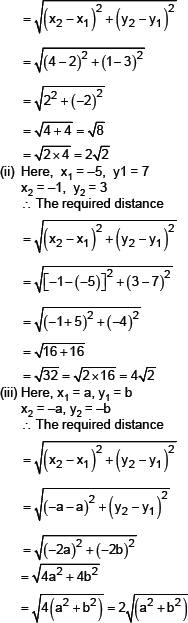# S.K.NEET

## Square and Square Roots

(i)   6
(ii)   14

#### Formulae used :

Distance Formula to find distance between two points (x1,y1) and (x2,y2) is :             D = √[(x2 – x1)2 + (y2 – y1)2 ]

#### Solution :

Sol. (i) Here x1 = 2, y1 = 3, x2 = 4 and y2 = 1
∴ The required distance(i)   6
(ii)   14

(i)   6
(ii)   14

(i)   6
(ii)   14

(i)   6
(ii)   14

(i)   6
(ii)   14

(i)   6
(ii)   14

(i)   6
(ii)   14

(i)   6
(ii)   14

(i)   6
(ii)   14

(i)   6
(ii)   14

(i)   6
(ii)   14

(i)   6
(ii)   14

(i)   6
(ii)   14